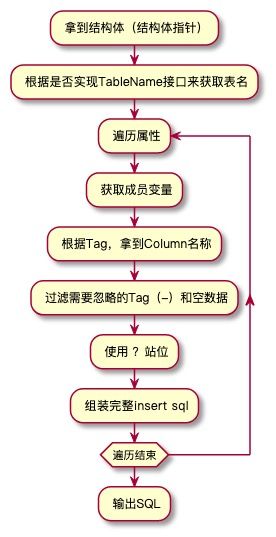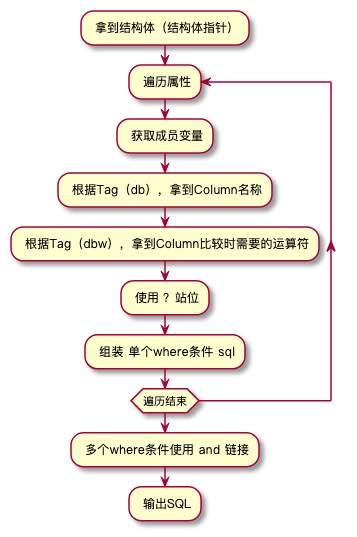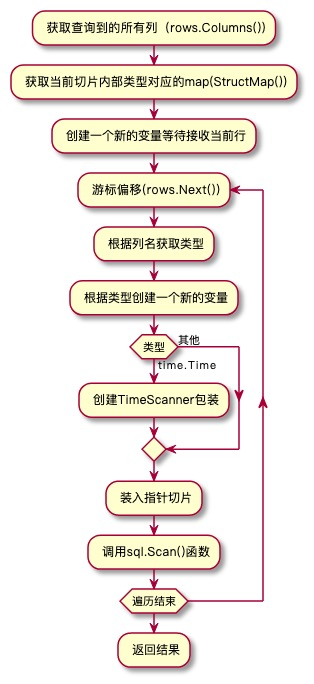# ORM浅谈

– 如何定制化一个自己的ORM

## 楔子

ORM框架 是我们开发过程中不可或缺的一环，无论是使用哪一种语言，都有相匹配的几款 ORM框架 供您挑选。

## 开始

### 设计

• SQL生成器

根据Map/Struct/Native 等方式生成sql

• 数据迭代器

将sql.Result进行迭代，封装后进行指针赋值

• 数据库特性及扩展

这部分相对就比较零散了，比如Tx等功能的支持，sql方言的处理，常用sql的工具封装，等等

### 准备工作 （Reflect）

``````//函数
reflect.ValueOf()//获取一个变量的值
reflect.TypeOf()//获取一个变量类型

//类型
reflect.Type//类型
reflect.Value//值
reflect.StructField//结构体成员变量
reflect.Tag//filed Tag

//结构体函数
Type.NumField()//获取成员变量的数量
Type.Field(i)  //获取成员变量的类型，返回值为reflect.StructField

Value.Kind() //获取类型
Value.Elem() //当类型为指针的时候，用于获取具体值

``````

### 准备工作 （SQL）

select语句因为存在聚合函数还有不同where条件组合等，并不太适合放在这里举例。

#### Insert

1. 按照列名插入

``````    INSERT INTO
table_name  (column1,column2,column3,...)
VALUES (value1,value2,value3,...);
``````
2. 提供全部数据插入

``````    INSERT INTO
table_name
VALUES (value1,value2,value3,...);
``````
3. set插入

``````    INSERT INTO table_name
SET
column1 = value1,
column2 = value2,
column3 = value3...;
``````

• 第二种SQL需要按顺序指定全部列的值
• 第三种SQL不能批量插入

#### Update

``````    UPDATE
table_name
SET
column1 = value1,
column2 = value2,
column3 = value3...
WHERE
field = xxx;
``````

## SQL生成器

``````    INSERT INTO
{表名}
({Field1 对应Column},{Field2 对应Column},{Field2 对应Column}...)
VALUES
({Value1},{Value2},{Value3}...)
``````

• 接口定义
``````    type(
Table interface {
TableName() string
}
)
``````
• 表名获取
``````    var tName string
if t, ok := i.(Table); ok {
//如果实现了接口，直接采用定制表名
tName = t.TableName()
} else {
//未实现接口，取struct名称的蛇形
tName = stringx.SnakeName(reflectx.StructName(i))
}
``````

1. 使用 `db:column_name` 来指定名称
2. 使用蛇形命名转换FieldName
3. 同时支持忽略属性
• 列名获取
``````        column := t.Tag.Get("db")
if len(column) == 0 {
//如无自定义column，取field名称的蛇形
column = stringx.SnakeName(t.Name)
}
// "-" 表示忽略，空数据 也直接跳过
if column == "-" || reflectx.IsNull(v) {
return nil
}
````````````    UPDATE
{表名}
SET
{Field1 对应Column} = value1,
{Field2 对应Column} = value2,
{Field3 对应Column} = value3...
WHERE
field = xxx;
``````

1. 自定义类型（type Cond Map[string]interface{}） – upper.io
2. 链式调用 sqlBuilder.Where(“a > ?",a).Where(“b = ?",b) – upper.io/GORM

``````const (
DbwTag         = "dbw"
DbwGreaterThan = "gt"
DbwLessThan    = "lt"
DbwEqual       = "eq"
)
``````

• 符号转换
``````func whereFlag(flag string) string {
switch flag {
case DbwEqual:
return "="
case DbwGreaterThan:
return ">"
case DbwLessThan:
return "<"
default:
panic("where flag " + flag + " not support")
}
}
``````
• 自动where语句的生成
``````func whereSql(t reflect.StructField) string {
where := t.Tag.Get(DbwTag)
if len(where) == 0 {
return ""
}
column := ColumnName(t) //这里参考之前写的根据Field获取ColumnName
return fmt.Sprintf("%s %s ?", column, whereFlag(where))
}
``````## 数据迭代器

• 列名获取
``````var rows *sql.Rows //由db.QueryRows()返回
columns, err := rows.Columns() //获取查询列名
``````

• 获取类型对应的结构
``````type Valuex struct {
reflect.Value
Tag reflect.StructTag
}
func StructMap(i interface{}) (res map[string]Valuex, err error) {
res = make(map[string]Valuex)
err = StructRange(i, func(t reflect.StructField, v reflect.Value) error {
res[ColumnName(t)] = Valuex{
Value: v,
Tag:   t.Tag,
}
return nil
})
return
}
``````

• 单次的数据查找
``````//fetchResult 通过列名抓取响应属性生成一个类型指针
func fetchResult(rows *sql.Rows, itemT reflect.Type, columns []string) (reflect.Value, error) {
objT := reflect.New(itemT)
values := make([]interface{}, len(columns))
fieldMap, _ := reflectx.StructMap(objT.Interface())
for i, k := range columns {
f, ok := fieldMap[k]
if !ok {
values[i] = new(interface{})
continue
}
switch curr.(type) {
case time.Time, *time.Time:
format := defTimeFormat
if dbFmt := f.Tag.Get("fmt"); len(dbFmt) > 0 {
format = dbFmt
}
values[i] = NewTimeScanner(f.Value, format)
default:
values[i] = curr
}
}
return objT, rows.Scan(values...)
}
``````

• Scanner接口实现
``````type (
TimeScanner struct {
v      reflect.Value
format string
}
)

const defTimeFormat = "2006-01-02 15:04:05"

func (t TimeScanner) Scan(src interface{}) (err error) {
var (
curr time.Time
)
switch src.(type) {
case []byte:
curr, err = time.Parse(t.format, string(src.([]byte)))
if err != nil {
return
}
case string:
curr, err = time.Parse(t.format, src.(string))
if err != nil {
return
}
case int64:
curr = time.Unix(src.(int64), 0)
case nil:
return
default:
return ErrTimeScan
}
currV := reflect.ValueOf(curr)
t.v.Set(currV)
return
}

func NewTimeScanner(v reflect.Value, format string) sql.Scanner {
return &TimeScanner{
v:      v,
format: format,
}
}
``````## 数据库特性及扩展

1. 兼容sql.DB和sql.Tx的接口封装
``````type (
executor struct {
exec SqlExecutor
}
)

func (d *executor) Exec(sql string, args ...interface{}) (sql.Result, error) {
return d.exec.Exec(sql, args...)
}

func (d *executor) Query(sql string, args ...interface{}) (*sql.Rows, error) {
return d.exec.Query(sql, args...)
}

func (d *executor) QueryRow(sql string, args ...interface{}) *sql.Row {
return d.exec.QueryRow(sql, args...)
}
``````

``````func (d *executor) Select(s ...string) Selector {
return NewSelector(d.exec, sqlbuilder.Select(s...))
}

func (d *executor) SelectFrom(s string) Selector {
return NewSelector(d.exec, sqlbuilder.SelectFrom(s))
}

func (d *executor) DeleteFrom(tableName string) Deleter {
return NewDeleter(d.exec, sqlbuilder.DeleteFrom(tableName))
}

func (d *executor) AutoInsert(t interface{}) (sql.Result, error) {
return NewInserter(d.exec, sqlbuilder.AutoInsert(t)).Exec()
}

func (d *executor) Count(i interface{}) (int64, error) {
var (
tb  = sqlbuilder.TableName(i)
sb  = sqlbuilder.Select("count(*) as c").From(tb)
tmp struct {
C int64
}
err error
)
err = NewSelector(d.exec, sb).AutoWhere(i).One(&tmp)
return tmp.C, err
}

func newExec(db SqlExecutor) *executor {
return &executor{exec: db}
}

``````

``````type (
Connector interface {
DriverName() string
ConnStr() string
GetPoolSize() int
}

MySQLConfig struct {
User     string `yaml:"user" json:"user" xml:"user"`
Pwd      string `yaml:"pwd" json:"pwd" xml:"pwd"`
Db       string `yaml:"db" json:"db" xml:"db"`
Host     string `yaml:"host" json:"host" xml:"host"`
Port     int    `yaml:"port" json:"port" xml:"port"`
PoolSize int    `yaml:"pool_size" json:"pool_size" xml:"pool_size"`
Charset  string `yaml:"charset" json:"charset" xml:"charset"`
Loc      string `yaml:"loc" json:"loc" xml:"loc"`
}
)

func (mc *MySQLConfig) DriverName() string {
return "mysql"
}

func (mc *MySQLConfig) ConnStr() string {
return fmt.Sprintf("%s:%s@tcp(%s:%d)/%s?charset=%s&parseTime=true&loc=%s", mc.User, mc.Pwd, mc.Host, mc.defPort(), mc.Db, mc.defCharset(), mc.defLoc())
}

func (mc *MySQLConfig) defPort() int {
if mc.Port == 0 {
mc.Port = 3306
}
return mc.Port
}

func (mc *MySQLConfig) GetPoolSize() int {
if mc.PoolSize == 0 {
mc.PoolSize = 10
}
return mc.PoolSize
}

func (mc *MySQLConfig) defCharset() string {
if len(mc.Charset) == 0 {
mc.Charset = "utf8"
}
return mc.Charset
}

func (mc *MySQLConfig) defLoc() string {
if len(mc.Loc) == 0 {
mc.Loc = "Asia%2FShanghai"
}
return mc.Loc
}

func New(conn db.Connector) (db.DataBase, error) {
if reflectx.IsNull(conn) {
return nil, errors.New("nil connector")
}
db, err := db.NewDB(conn.DriverName(), conn.ConnStr())
if err == nil {
db.SetMaxOpenConns(conn.GetPoolSize())
}
return db, err
}
``````

``````    cfg := &borm.MySQLConfig{
User: "root",
Pwd:  "123456",
Db:   "test",
Host: "127.0.0.1",
}

db, err := borm.New(cfg)
if err != nil {
panic(err)
}
``````

## 后记